Virus evolution and population genetics

Richard Neher
Biozentrum, University of Basel

Viruses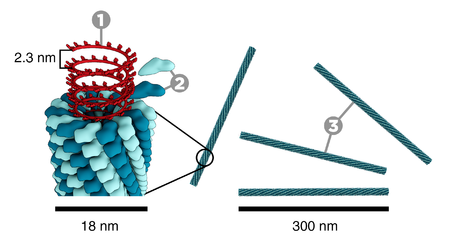tobacco mosaic virus (Thomas Splettstoesser, wikipedia)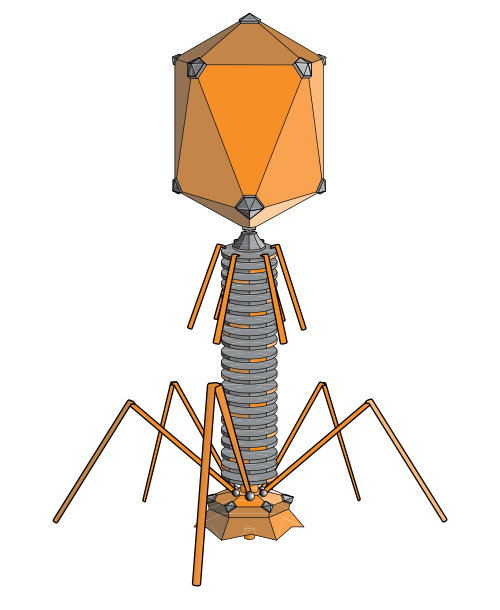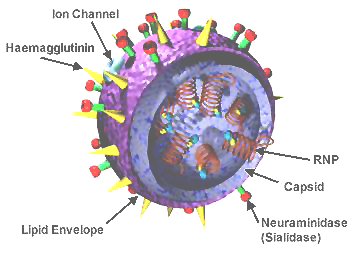influenza virus wikipediahuman immunodeficiency virus wikipedia
• rely on host to replicate
• little more than genome + capsid
• genomes typically 5-200k bases (+exceptions)
• most abundant organisms on earth $\sim 10^{31}$

Evolution of HIV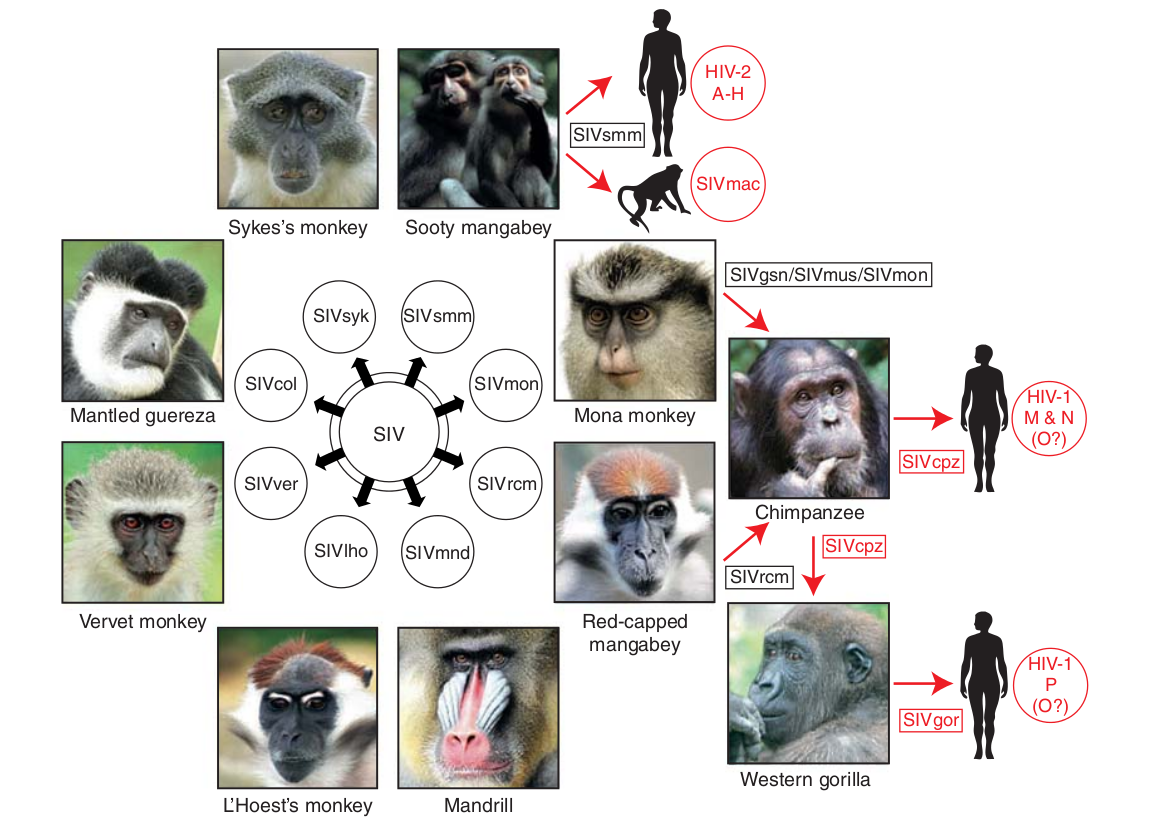• Chimp → human transmission around 1900 gave rise to HIV-1 group M
• ~100 million infected people since
• subtypes differ at 10-20% of their genome
• HIV-1 evolves ~0.1% per year
image: Sharp and Hahn, CSH Persp. Med.

HIV infection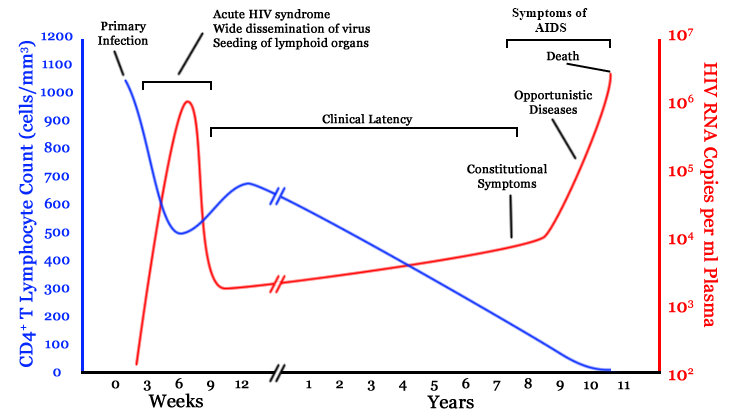• $10^8$ cells are infected every day
• the virus repeatedly escapes immune recognition
• integrates into T-cells as latent provirus
image: wikipedia

Some viruses evolve a million times faster than animals

Animal haemoglobin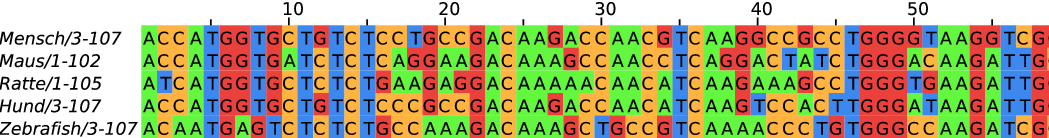HIV protein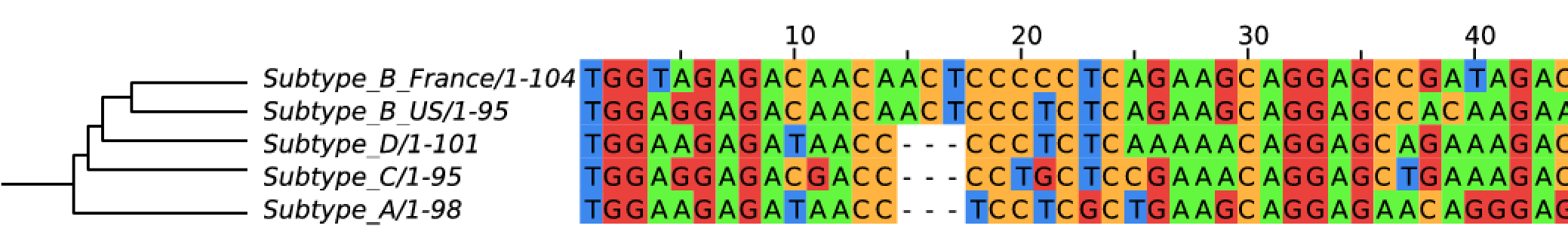Development of sequencing technologies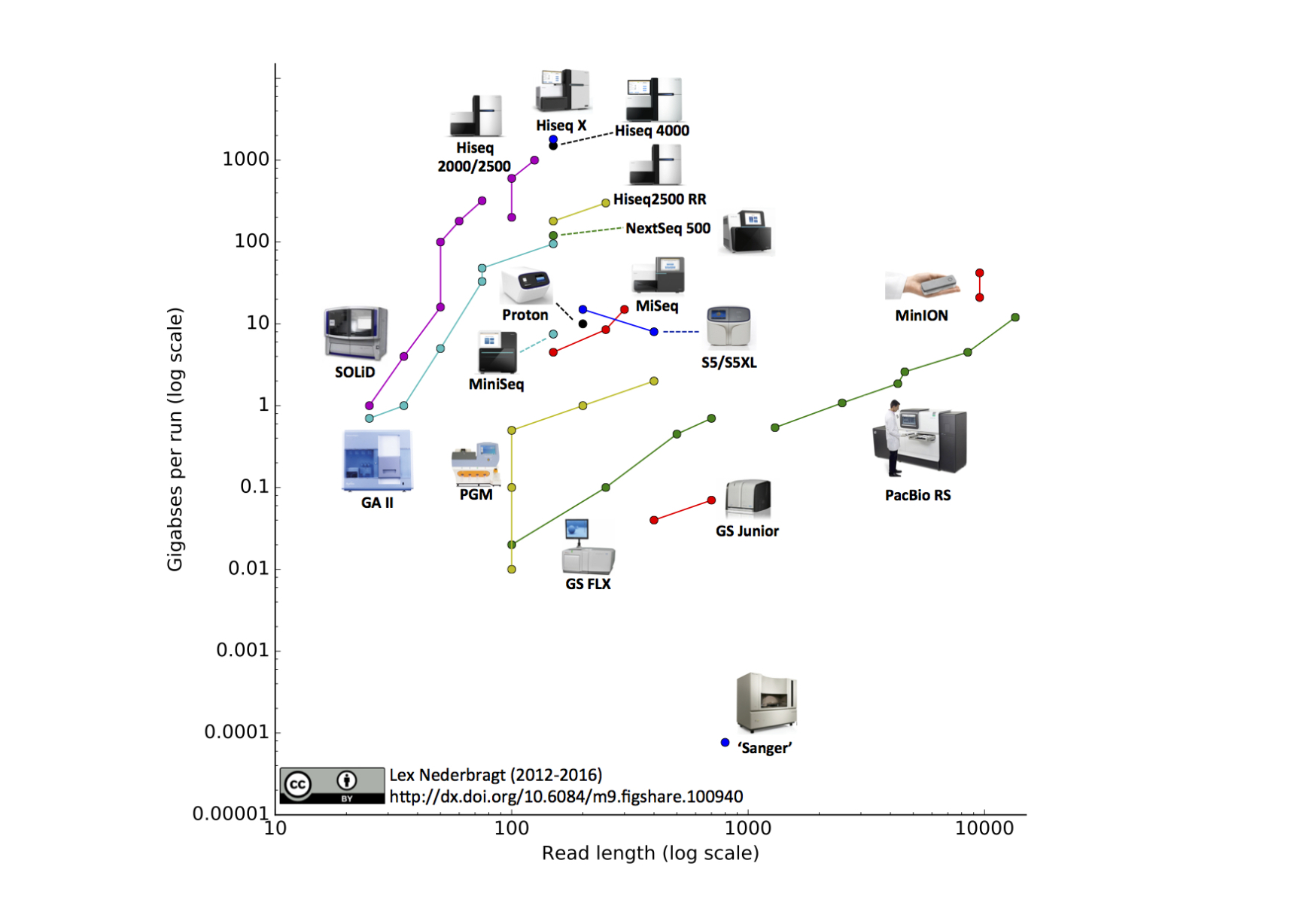We can now sequence...
• thousands of bacterial isolates
• thousands of single cells
• populations of viruses, bacteria or flies
• diverse ecosystems

HIV-1 evolution within one individual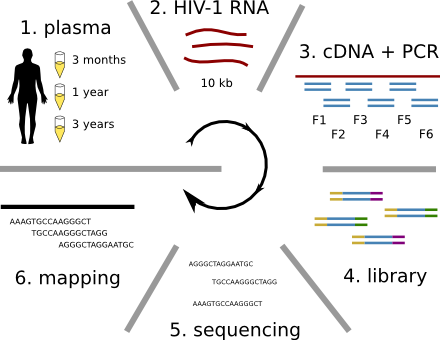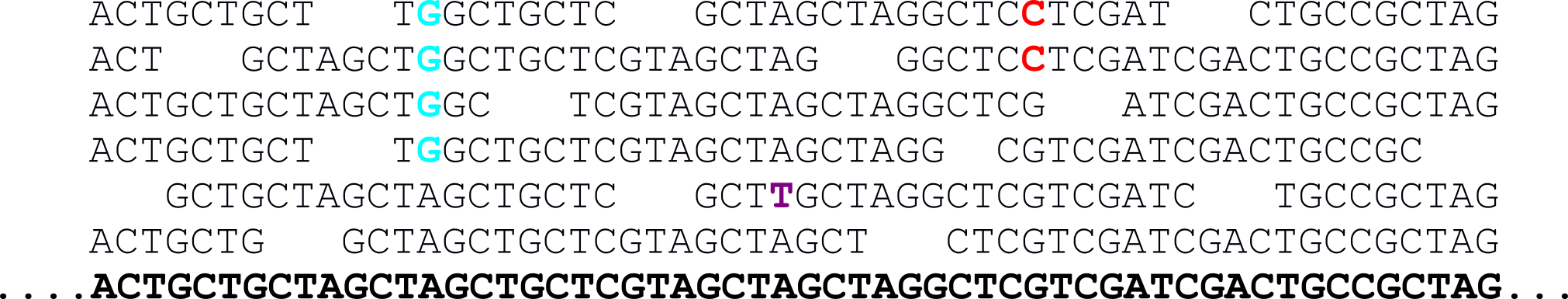silouhette: clipartfest.com, Zanini at al, 2015. Collaboration with Jan Albert and his group

Immune escape in early HIV infection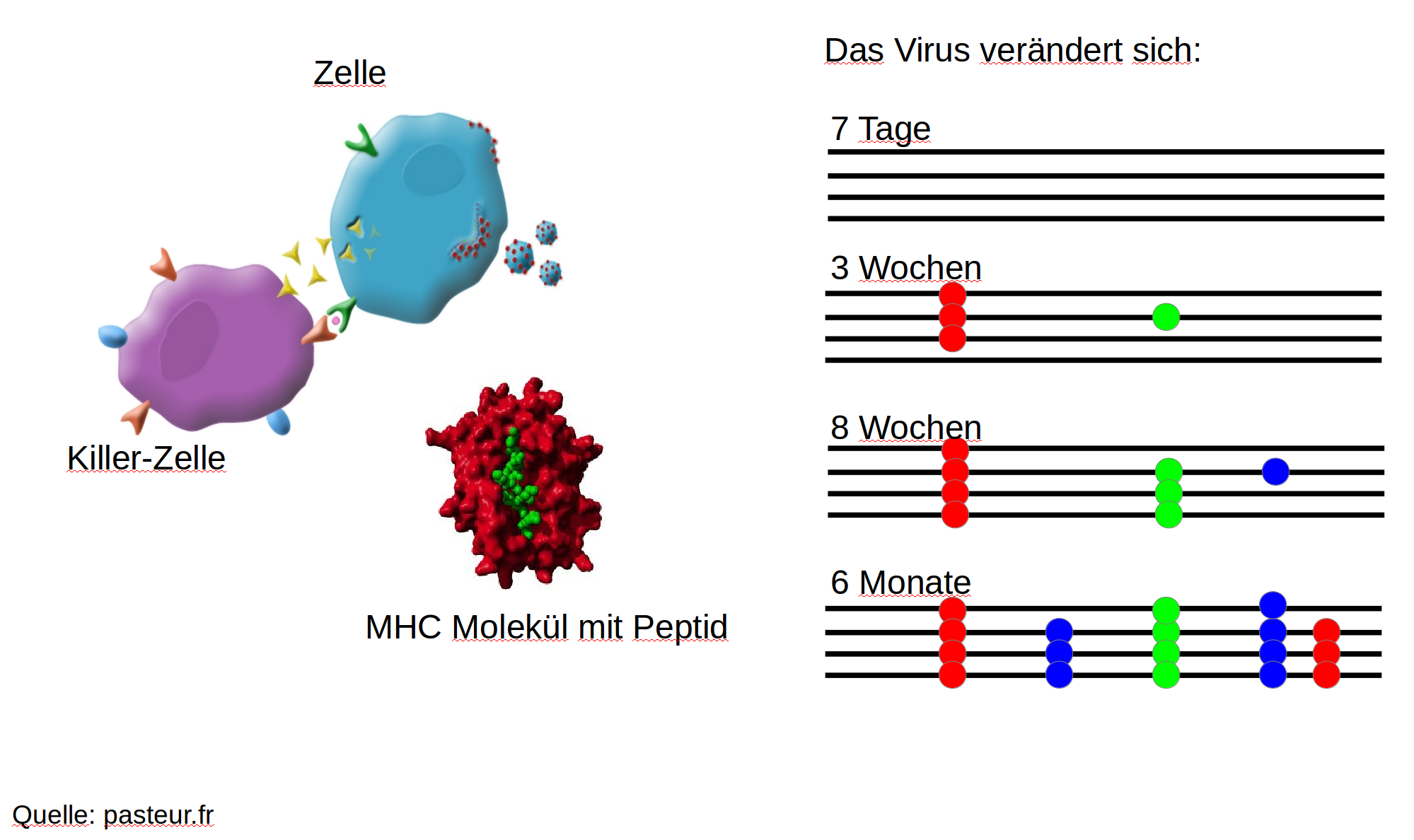Immune escape in early HIV infection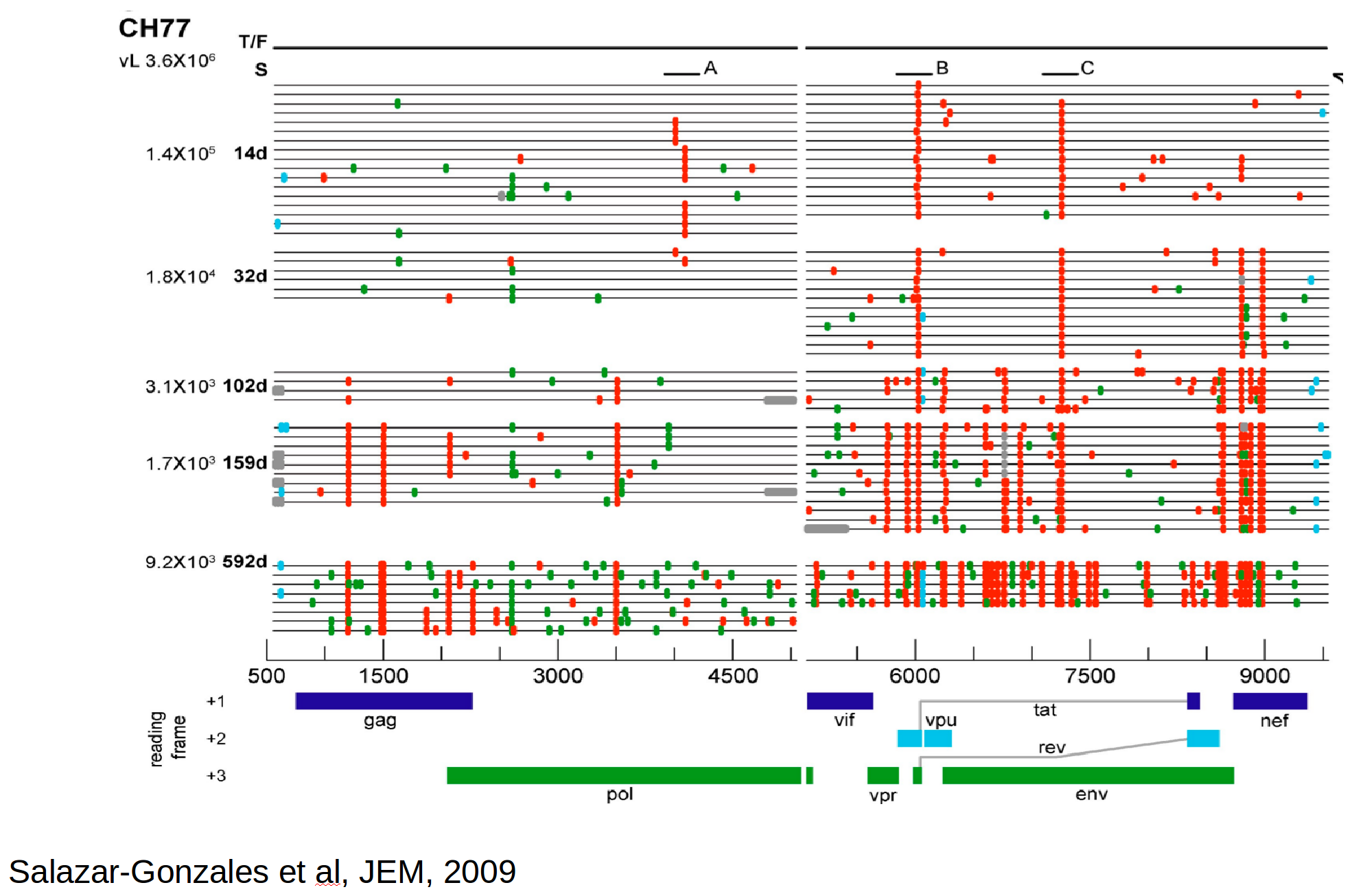Population genetics & evolutionary dynamicsSelective sweeps

• Viruses carrying a beneficial mutation have more offspring: on average $1+s$ instead of $1$
• $s$ is called selection coefficient
• Fraction $x$ of viruses carrying the mutation changes as $$x(t+1) = \frac{(1+s)x(t)}{(1+s)x(t) + (1-x(t))}$$
• In continuous time → logistic differential equation: $$\frac{dx}{dt} = sx(1-x) \Rightarrow x(t) = \frac{e^{s(t-t_0)}}{1+ e^{s(t-t_0)}}$$

Mutation rates and diversity and neutral sitesZanini et al, Virus Evolution, 2017

Balance between mutation and deleterious mutations

• mutation away from preferred state with rate $\mu$
• selection against non-preferred state with strength $s$
• variant frequency dynamics: $\frac{d x}{dt} = \mu -s x$
• equilibrium frequency: $\bar{x} = \mu/s$
• fitness cost: $s = \mu/\bar{x}$

Time-scaled phylogenies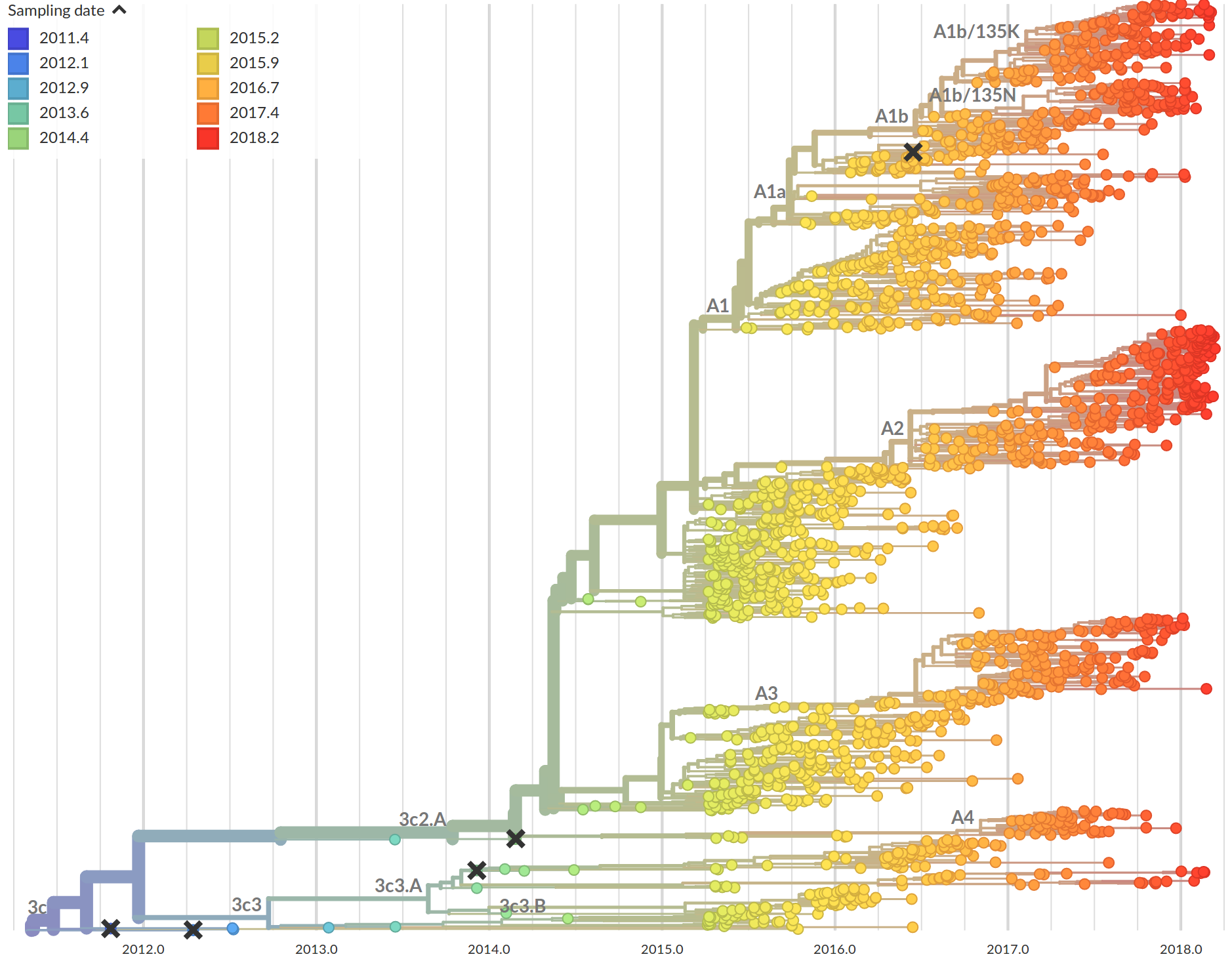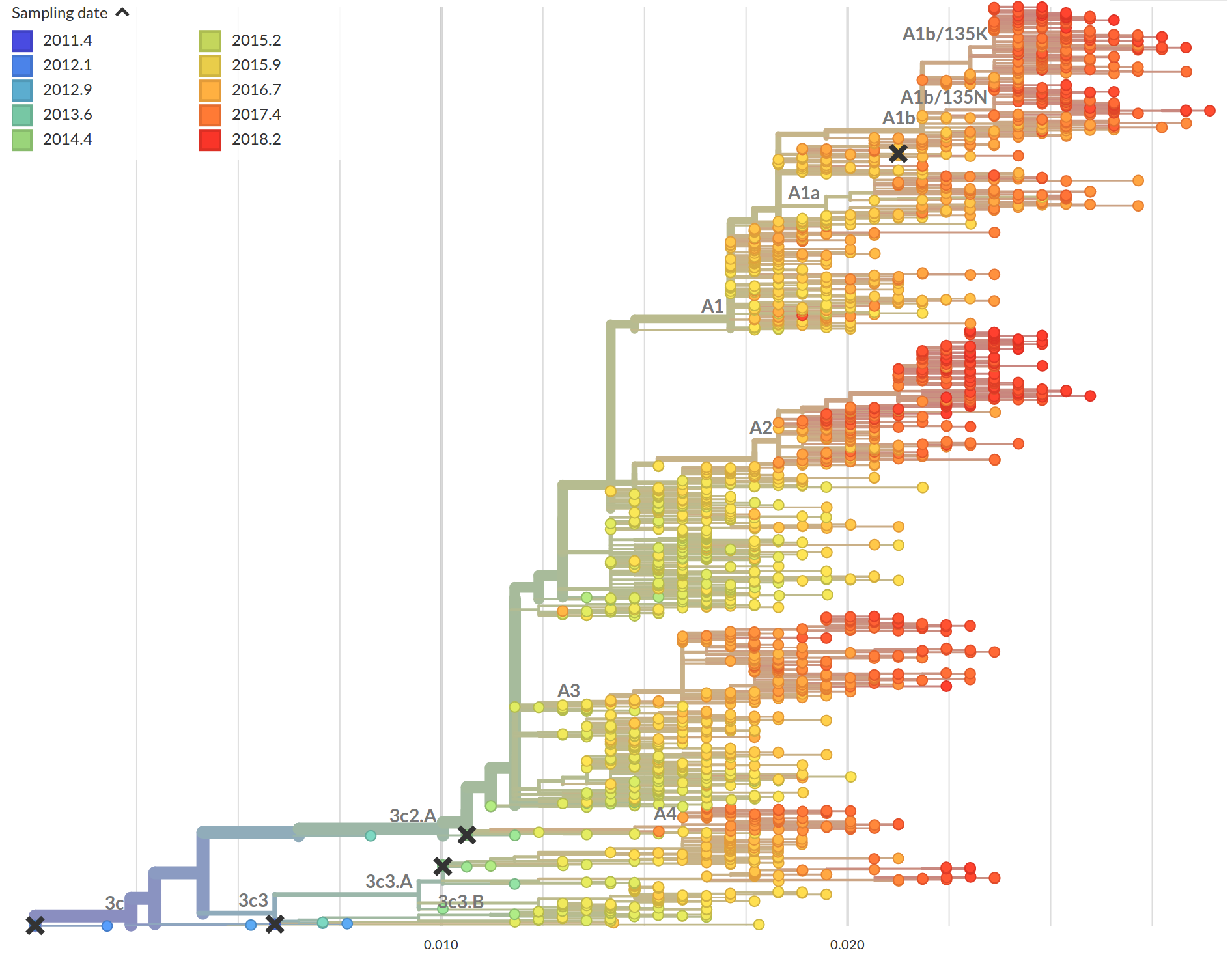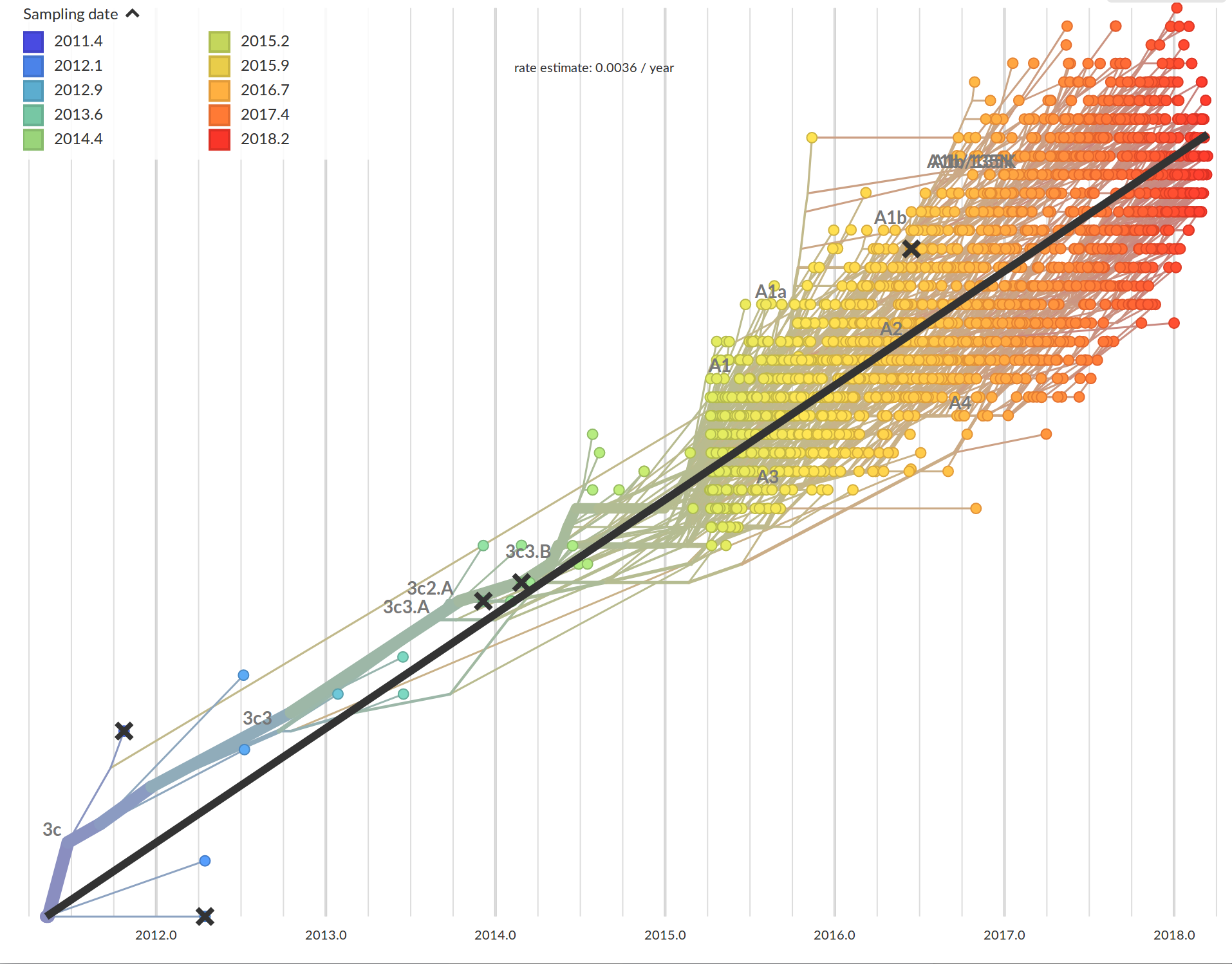Tree building optimization with temporal constraints

• Time stamps single out a root
• Root can be found by optimizing root-to-tip regression
• BEAST: Markov-Chain Monte Carlo tree sampler
• If topology is correct, temporal constraints can be accounted for in linear time
• Multiple tools: treedate, LSD, treetime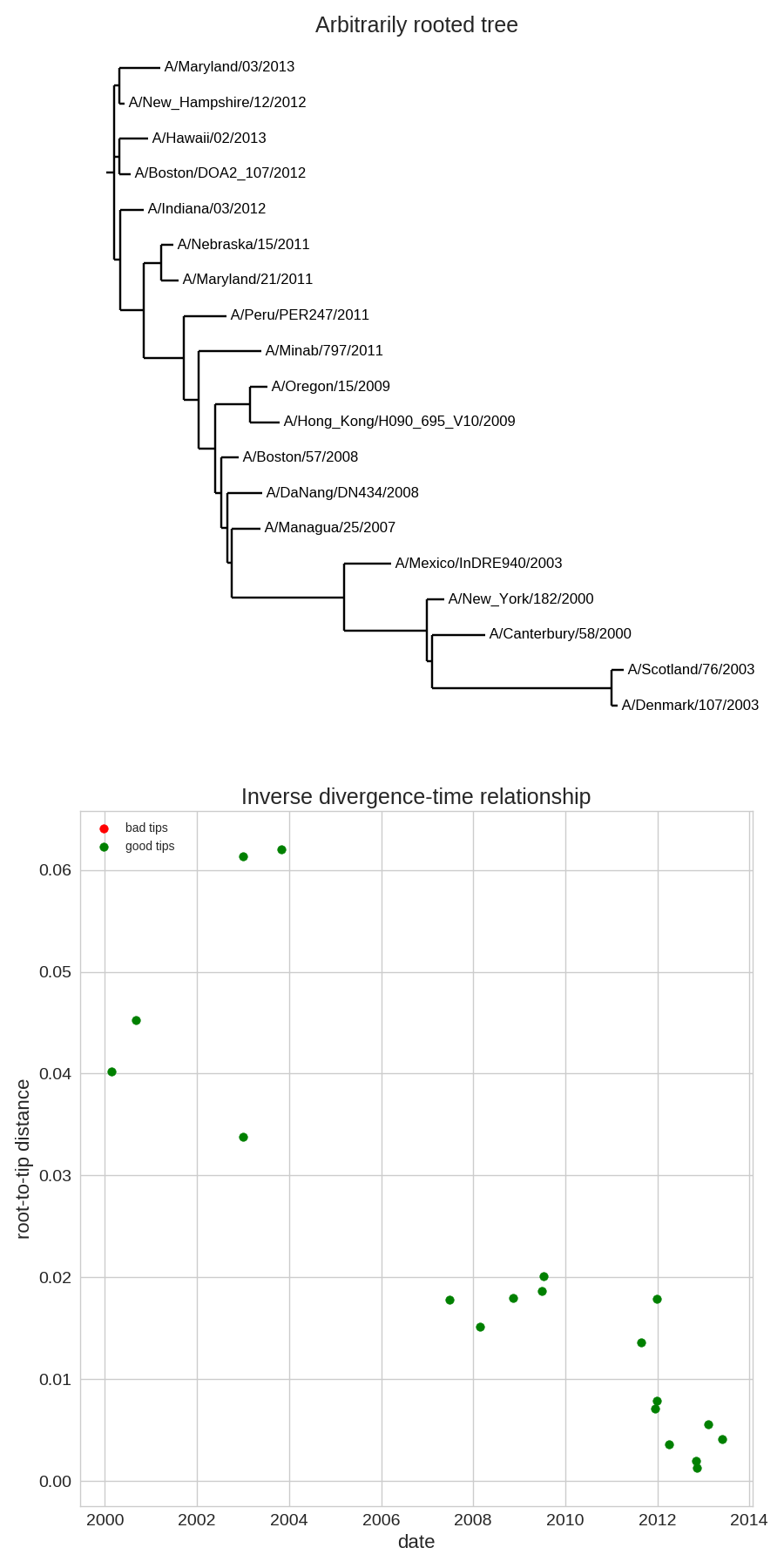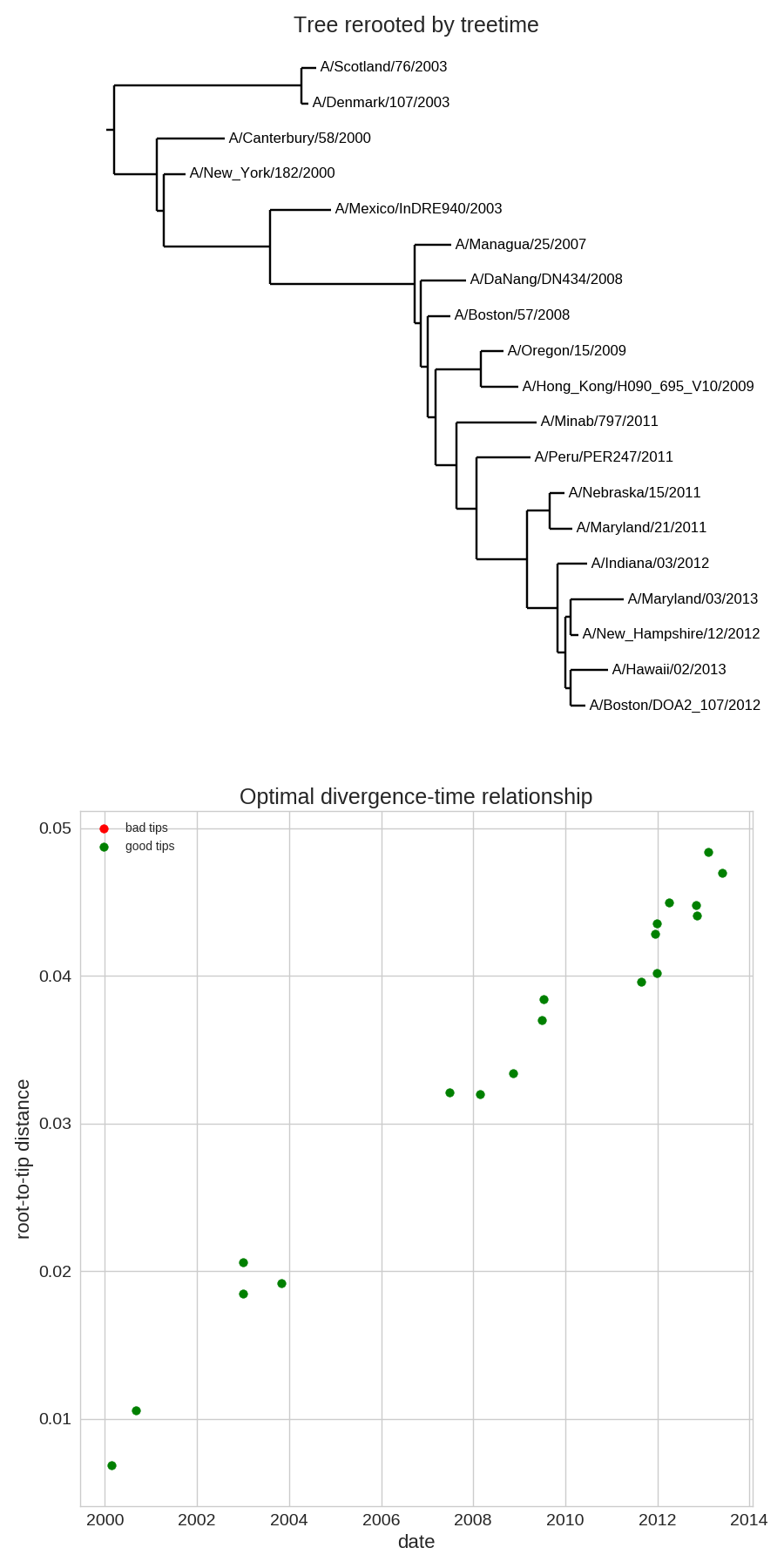Time-scaled phylogenies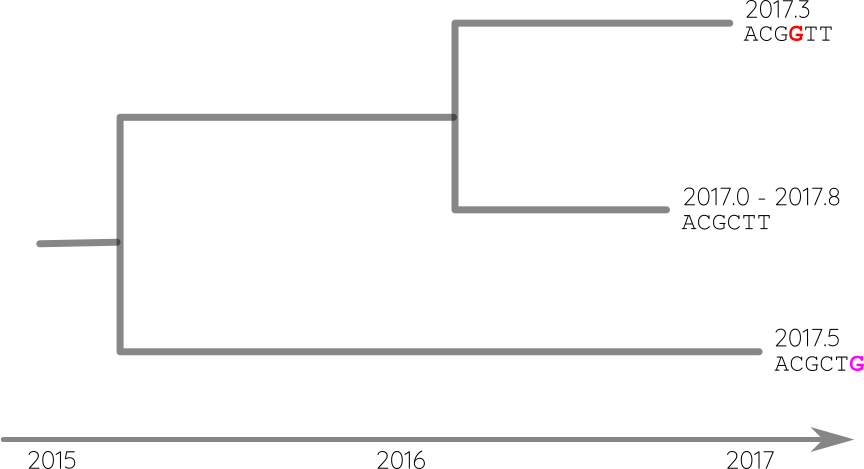Attach sequences and dates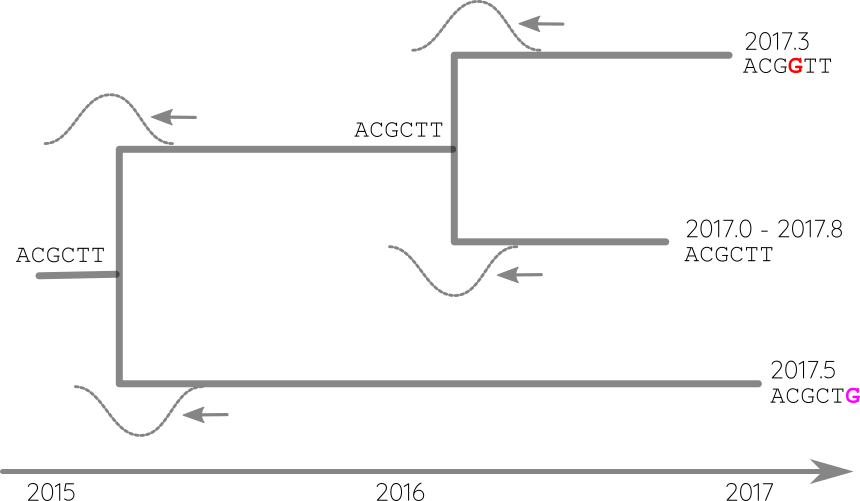Propagate temporal constraints via convolutions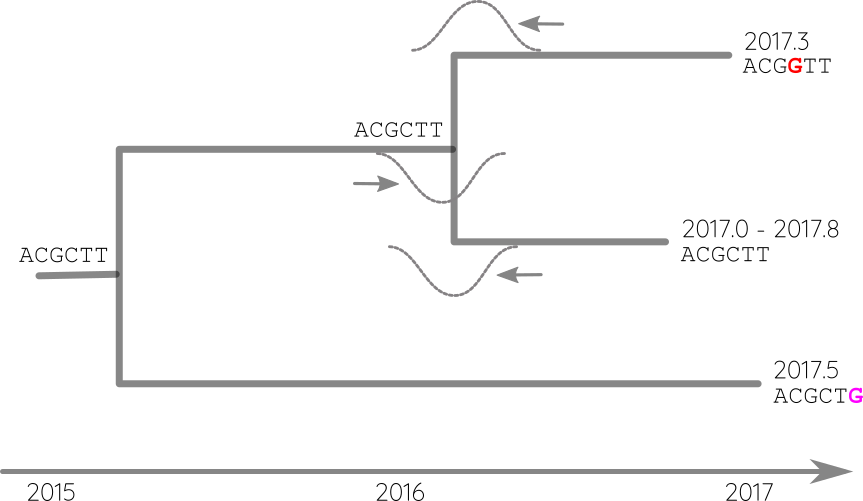Time-scaled phylogenies

• Calibration points can be longitudinal samples, ancient DNA or fossils
• Rates can vary between proteins and organisms from 0.01/year to $<10^{-8}$/y
• Some site change often, some rarely → saturation
• The apparent rate changes over time
• Divergence times are often under estimated.